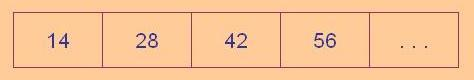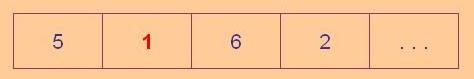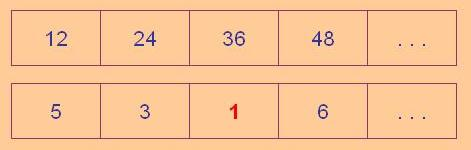#### You may also like### N000ughty Thoughts

How many noughts are at the end of these giant numbers?### DOTS Division

Take any pair of two digit numbers x=ab and y=cd where, without loss of generality, ab > cd . Form two 4 digit numbers r=abcd and s=cdab and calculate: {r^2 - s^2} /{x^2 - y^2}.### Mod 3

Prove that if a^2+b^2 is a multiple of 3 then both a and b are multiples of 3.

# There's Always One Isn't There

##### Age 14 to 16Challenge Level

Take any pair of numbers, say 9 and 14.

Take the larger number, 14, and count up by that amount :Then divide each of the values by 9, your chosen smaller number, and look at the remainders.#### Notice there's a one.

Now do the same again but using different numbers, say 7 and 12.

Counting in twelves and dividing each result by 7 :#### Again somewhere in those remainders is a one.

Pick the pairs how you like, somewhere there'll always be a one - won't there?

What actually happens?

Why?